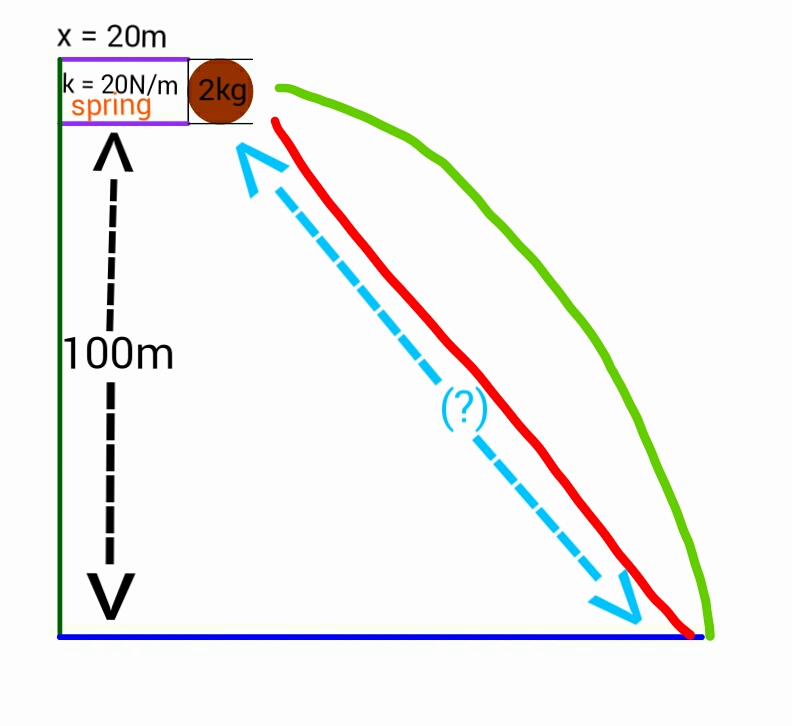# Horizontal pinballA ball of mass $2 \text{ kg}$ is placed in front of a spring having constant $20 \text{ N/m}$ and compression $20 \text{ m}$ at a height of $100 \text{ m}$ above the ground.

Now, the spring is released, giving the ball a horizontal velocity.

Find the total displacement (in meters) of the ball from the spring when it hits the ground.

Details and Assumptions:

• The ball does not rebound after hitting the ground first.
• Air friction is negligible.
• The spring is 100% efficient.
• Take $g=10 {\text{ m/s}}^2$ as the acceleration due to gravity.
×